Home > A2C > Chapter 7 > Lesson 7.3.1 > Problem7-163

7-163.
1. Consider the function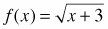. Homework Help ✎

1. What are the domain and range of f(x)?

2. If g(x) = x − 10, what is f(g(x))?

3. What are the domain and range of f(g(x))?

4. Is f(g(x)) = g(f(x))? Justify why or why not.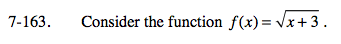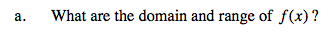Think about what the graph would look like.
Try making a table if you do not remember what the graph looks like.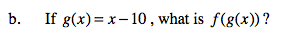f(g(x)) is the same as f(x − 10). Why?
Find f(x − 10).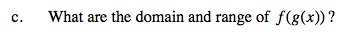See the hint in part (a).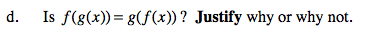f(g(x)) is the same as f(x − 10).

$g(f(x)) \text{ is the same as } g\left(\sqrt{(x + 3)}\right).$

Will these yield the same function?